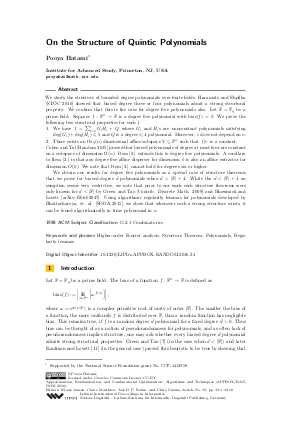Document# On the Structure of Quintic Polynomials

### Author Pooya Hatami## File

LIPIcs.APPROX-RANDOM.2016.33.pdf
• Filesize: 0.49 MB
• 18 pages

## Cite As

Pooya Hatami. On the Structure of Quintic Polynomials. In Approximation, Randomization, and Combinatorial Optimization. Algorithms and Techniques (APPROX/RANDOM 2016). Leibniz International Proceedings in Informatics (LIPIcs), Volume 60, pp. 33:1-33:18, Schloss Dagstuhl - Leibniz-Zentrum für Informatik (2016)
https://doi.org/10.4230/LIPIcs.APPROX-RANDOM.2016.33

## Abstract

We study the structure of bounded degree polynomials over finite fields. Haramaty and Shpilka [STOC 2010] showed that biased degree three or four polynomials admit a strong structural property. We confirm that this is the case for degree five polynomials also. Let F=F_q be a prime field. Suppose f:F^n to F is a degree five polynomial with bias(f)=delta. We prove the following two structural properties for such f. 1. We have f= sum_{i=1}^{c} G_i H_i + Q, where G_i and H_is are nonconstant polynomials satisfying deg(G_i)+deg(H_i)<= 5 and Q is a degree <5 polynomial. Moreover, c does not depend on n. 2. There exists an Omega_{delta,q}(n) dimensional affine subspace V subseteq F^n such that f|_V is a constant. Cohen and Tal [Random 2015] proved that biased polynomials of degree at most four are constant on a subspace of dimension Omega(n). Item 2.]extends this to degree five polynomials. A corollary to Item 2. is that any degree five affine disperser for dimension k is also an affine extractor for dimension O(k). We note that Item 2. cannot hold for degrees six or higher. We obtain our results for degree five polynomials as a special case of structure theorems that we prove for biased degree d polynomials when d<|\F|+4. While the d<|F|+4 assumption seems very restrictive, we note that prior to our work such structure theorems were only known for d<|\F| by Green and Tao [Contrib. Discrete Math. 2009] and Bhowmick and Lovett [arXiv:1506.02047]. Using algorithmic regularity lemmas for polynomials developed by Bhattacharyya, et al. [SODA 2015], we show that whenever such a strong structure exists, it can be found algorithmically in time polynomial in n.
##### Keywords
• Higher-order Fourier analysis
• Structure Theorem
• Polynomials
• Regularity lemmas

## Metrics

• Access Statistics
• Total Accesses (updated on a weekly basis)
0

## References

1. Arnab Bhattacharyya, Eldar Fischer, Hamed Hatami, Pooya Hatami, and Shachar Lovett. Every locally characterized affine-invariant property is testable. In Proceedings of the 45th annual ACM symposium on Symposium on theory of computing, STOC'13, pages 429-436, New York, NY, USA, 2013. ACM. URL: http://dx.doi.org/10.1145/2488608.2488662.
2. Arnab Bhattacharyya, Pooya Hatami, and Madhur Tulsiani. Algorithmic regularity for polynomials and applications. In Proceedings of the Twenty-Sixth Annual ACM-SIAM Symposium on Discrete Algorithms, SODA 2015, San Diego, CA, USA, January 4-6, 2015, pages 1870-1889, 2015. URL: http://dx.doi.org/10.1137/1.9781611973730.125.
3. Abhishek Bhowmick and Shachar Lovett. Bias vs structure of polynomials in large fields, and applications in effective algebraic geometry and coding theory. CoRR, abs/1506.02047, 2015. URL: http://arxiv.org/abs/1506.02047.
4. Abhishek Bhowmick and Shachar Lovett. The list decoding radius of reed-muller codes over small fields. In Proceedings of the Forty-Seventh Annual ACM on Symposium on Theory of Computing, STOC'15, pages 277-285, New York, NY, USA, 2015. ACM. URL: http://dx.doi.org/10.1145/2746539.2746543.
5. Gil Cohen and Avishay Tal. Two structural results for low degree polynomials and applications. RANDOM, 2015.6. Leonard Eugene Dickson. Linear groups: With an exposition of the Galois field theory. with an introduction by W. Magnus. Dover Publications, Inc., New York, 1958.7. Ben Green and Terence Tao. The distribution of polynomials over finite fields, with applications to the Gowers norms. Contrib. Discrete Math., 4(2):1-36, 2009.8. Elad Haramaty and Amir Shpilka. On the structure of cubic and quartic polynomials. In STOC'10 - Proceedings of the 2010 ACM International Symposium on Theory of Computing, pages 331-340. ACM, New York, 2010.9. Hamed Hatami, Pooya Hatami, and James Hirst. Limits of Boolean functions on 𝔽ⁿ_p. Electron. J. Combin., 21(4):Paper 4.2, 15, 2014.10. Hamed Hatami, Pooya Hatami, and Shachar Lovett. General systems of linear forms: equidistribution and true complexity. Advances in Mathematics, 292:446-477, 2016.11. Tali Kaufman and Shachar Lovett. Worst case to average case reductions for polynomials. Foundations of Computer Science, IEEE Annual Symposium on, 0:166-175, 2008. URL: http://dx.doi.org/10.1109/FOCS.2008.17.
12. Tali Kaufman, Shachar Lovett, and Ely Porat. Weight distribution and list-decoding size of reed-muller codes. Information Theory, IEEE Transactions on, 58(5):2689-2696, 2012.13. Rudolf Lidl and Harald Niederreiter. Introduction to finite fields and their applications. Cambridge university press, 1994.14. Shachar Lovett, Roy Meshulam, and Alex Samorodnitsky. Inverse conjecture for the Gowers norm is false. Theory Comput., 7:131-145, 2011. URL: http://dx.doi.org/10.4086/toc.2011.v007a009.
15. Tom Sanders. Additive structures in sumsets. Math. Proc. Cambridge Philos. Soc., 144(2):289-316, 2008. URL: http://dx.doi.org/10.1017/S030500410700093X.
16. Terence Tao and Tamar Ziegler. The inverse conjecture for the Gowers norm over finite fields in low characteristic. Ann. Comb., 16(1):121-188, 2012. URL: http://dx.doi.org/10.1007/s00026-011-0124-3.
X

Feedback for Dagstuhl Publishing